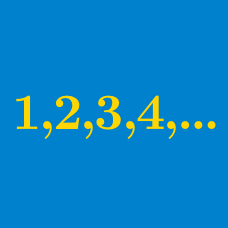Algebra

# Arithmetic Progressions: Level 1 ChallengesAs a young boy, Gauss was able to calculate math quickly. He was asked for the value of

$1 +2 + 3 + \cdots + 100.$

Hint: $1 + 2 + 3 + \cdots + n = \frac 12 n(n+1)$.

###### Image Source: Wikipedia.

An arithmetic sequence has 11 terms which sum to 220. What is the middle (6th) term in the sequence?What is the value of $n$ in the following expression?

$1 + 2 + 3 + \dots + (n-1) + n + (n-1) + \dots + 3 + 2 + 1 = 289$

###### Image Credit: Ricardo Liberato, CC SA 2.0

In an arithmetic progression of 200 terms,
the 27th term equals to 2,
the 174th term equals to 4.

Find the sum of all the terms of the arithmetic progression.

$\large 100+99+98+97+96+\cdots + 50 = \ ?$

×